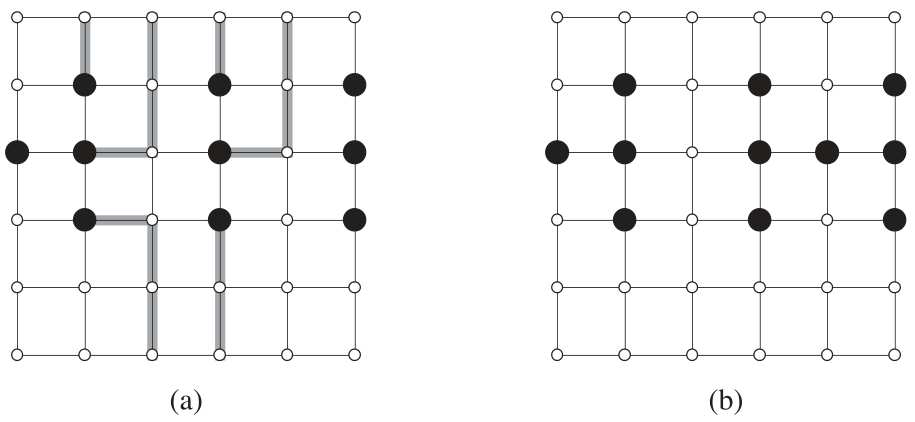# P2691 逃离

• 30通过
• 116提交
• 题目提供者 洛谷
• 评测方式 云端评测
• 标签 图论
• 难度 提高+/省选-
• 时空限制 1000ms / 128MB
• 提示：收藏到任务计划后，可在首页查看。

## 题目描述

译自 CLRS Problem 26-1 Escape problem

在一个 $n\times n$ 的网格中有 $m$ 个起始点 $(x_1, y_1),$ $(x_2, y_2),$ $\dots,$ $(x_m, y_m)$，试问：能否为这些结点分别找一条到边界的路径，且这 $m$ 条路径互不相交（即任意两条路径上没有一个相同的结点）。黑点表示起始点，其他点用白点表示。找出的路径用加粗的线表示。图 (a) 存在符合条件的 $m$ 条路径，图 (b) 则不存在。

## 输入输出格式

输入格式：

第一行是一个整数，为 $n$ $(1\le n≤35)$。

第二行还是一个整数，为 $m(1\le m\le n^2)$。

以下 $m$ 行，第 $(i+2)$ 行包含两个整数 $x_i$ 和 $y_i$，表示第 $i$ 行第 $j$ 列的点是起始点。保证起始点坐标互不相同。

输出格式：

只包括一行。若存在逃脱输出 YES，不存在逃脱输出 NO

## 输入输出样例

输入样例#1： 复制
6
10
2 2
2 4
2 6
3 1
3 2
3 4
3 6
4 2
4 4
4 6

输出样例#1： 复制
YES
提示
标程仅供做题后或实在无思路时参考。
请自觉、自律地使用该功能并请对自己的学习负责。
如果发现恶意抄袭标程，将按照I类违反进行处理。